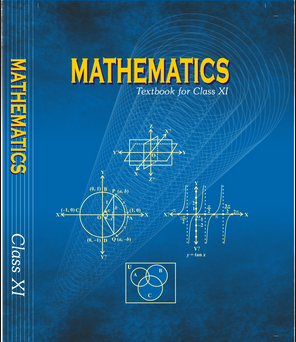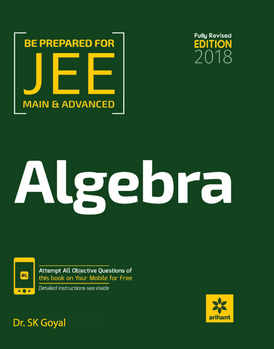# Sequence and series   Share

## What is Sequence and series

Sequences and Series

Algebra at the JEE level is very interesting. All topics are more or less independent of each other. And one of the interesting and important topics is Sequences and Series and every year you will get 1 - 2 question in JEE Main exam as well as in other engineering entrance exams. JEE question paper is highly unpredictable, you never know questions from which topic will be asked. A general trend noticed in Mathematics paper is that a question involving multiple concepts are asked. For instance, you will find that questions from Calculus, Matrices and Determinant and Functions where concepts of Sequences and Series are involved. As compared to other chapters in maths, Sequences and Series requires less effort to prepare for the examination.

## Why Sequences and Series

There was a con man who made chessboards for the emperor. The craftsman was good at his work as well as with his mind. He knew that the emperor loved chess. So he conspires a plan to trick the emperor to give him a large amount of fortune. When the craftsman presented his chessboard at court, the emperor was so impressed by the chessboard, that he said to the craftsman

Prepare Online for JEE Main/NEET

Crack JEE 2021 with JEE/NEET Online Preparation Program

The craftsman responded

"Your Highness, I don't want money for this. Or jewels. my wish was simple.  All I want is a little rice."

The emperor agreed, amazed that the man had asked for such a small reward

"I've got rice. How much rice?"

"All I want," said the craftsman, "is for you to put a single grain of rice on the first square, two grains on the second square, four on the third square, eight on the fourth square, and so on and so on  for all 64 squares, with each square having double the number of grains as the square before."

"Well, I can do that," said the emperor, not thinking much. And he ordered his treasurer to pay the craftsman for the chessboard.Well, that turned out to be more than a little difficult. The first few squares on the board cost the emperor 1 grain, then 2, then 4 ... by the end of the first row, he was up to 128 grains.

In the second row, things got out of control. By the 21st square he owed over a million grains of rice; by the 41st, it was over a trillion grains of rice — more rice than he, his subjects or any emperor anywhere could afford in the world.

After all, he was the emperor. He knew how to handle such situations

"I will pay you," he told the craftsman. "But before you receive the grains of rice, just to be sure you are getting what you asked for, I'd like you to count each and every grain I give you."

"Oh, that won't be required," said the craftsman.

"Oh, it is necessary," said the emperor. "I wouldn't want to cheat you."

So now you tell me, What will be the total number of grains? How much time does craftsman require to complete the count? The amount of rice that craftsman asked, will that be available on our planet?

Well, all the answers to these questions you will able to tell when you study Sequences and Series.

After reading this chapter  you will be able to:

• Write the first few terms of a sequence

• Find a formula for the general term (nth term) of a sequence

• Find the sum and partial sum

• Use of summation notation to write a sum

## Important Topics

• Sequences

• Arithmetic and Geometric Progression

• Arithmetic and Geometric Mean

• Harmonic Progression

• Sum up to n terms

• Arithmetic-geometric series

## Overview of Chapter- Sequence and Series

Sequences

A sequence is an arrangement of a list of objects or numbers in a definite order. The numbers or objects are also known as the terms of the sequence. A sequence containing a finite number of terms is called a finite sequence and a sequence is called infinite if it is not a finite sequence.

eg.. 2, 4, 6, 8, 10, 12, …..

Often when working with sequences we do not want to write out all the terms. We want a more compact way to show how each term is defined. And hence, we define the general term and it is denoted by an.or tn.

For the above example, an = 2n, where n is Integer.

Arithmetic Progression

An arithmetic progression is a sequence whose terms increase or decrease by a fixed number. The fixed number is called a common difference (d) of the AP. If a is the first term,  and d is a common difference, then AP can be written as

a, a + d, a + 2d, a + 3d,   ……….. a + (n - 1)d

Where a + (n - 1)d is the general term of an AP.

Sum of n terms of an AP is given by

You know that the sum of the interior angle is 1800, of a quadrilateral, is 3600 and of a Pentagon is 5400 . Assume that the patterns continue. Then the sum of the interior angle of an Octagon (8 sided ) isThe pattern 1800, 3600, 5400 …….is arithmetic with common difference 1800. The 8-sided figure will be the 6th term in the sequance.

6th term is

a6 = a1 + (n - 1)(d)

a6 = 1800 + (6 - 1)(1800) = 10800Geometric Progression

A geometric sequence is a sequence such that if the ratio of any term and its just preceding term is constant throughout. The onstant called the common ratio which is denoted by r.

Where,

r = common ratio

a1 = first term

a2 = second term

an = nth term

Important Formula of Geometric Progression

1. The general term of a GP is an = arn-1
2. Sum of n-term of a geometric progression is given by
3. If geometric progression is infinite and common ratio, -1 < r < 1, then the sum of the series is given by

Now, consider the earlier storyThe first square contains 1 rice, the second square contains 2 rice grains, the 3rd square contains 4 rice grains, 4th one contains 8 rice grains and so on…

The sequence will be like 1, 2, 4, 8, 16, 32,......... or {20, 21, 23, 24……..263}, this is a geometric progression,

Using the formula of summation of a geometric progression

Which is weighing about 1,199,000,000,000 metric tons (assuming 65 mg as the mass of one grain of rice)

It would have taken the craftsman a half-trillion years, about 42 times the age of our universe, to complete his count.

## How to prepare Sequences and Series?

Sequences and series is one of the easiest topics, you can prepare this topic without applying many efforts

• Start with basic theory, understand all the definition of the Sequences, series, and Arithmetic and geometric progression.

• Derive and understand the formulae of General Term, Sum of the Series of n terms and remember standard results.

• Learn the concept behind Harmonic sequences and general term of Harmonic sequences.

• Derive all the formulae of summation of some special series like the sum of first n natural number, the summation of odd numbers, sum of cube of first n natural numbers, etc.

• Mean is one of the most important concepts, as AM-GM is used to determine the minimum and maximum value of the function.

• After the study, each concept, do a lot of solved examples in order to comprehend the concept as well as their applications.

• Make sure that after studying certain section/concept, solve questions related to those concepts without looking into the solutions and practice MCQ from the above-mentioned books and solve all the previous year problems asked in JEE.

• Don’t let any doubt remain in your mind and clear all the doubts with your teachers or with your friends.

## Best Books For Preparation:-

First, finish all the concept, example and question given in NCERT Maths Book. You must thorough with the theory of NCERT. Then you can refer to the book Cengage Mathematics Algebra. Sequences and Series are explained very well in this book and there are ample amount of questions with crystal clear concepts. You can also refer to the book Arihant Algebra by SK Goyal or RD Sharma. But again the choice of reference book depends on person to person, find the book that best suits you the best depending on how well you are clear with the concepts and the difficulty of the questions you require.

## Maths Chapter-wise Notes for Engineering exams

 Chapters Chapters Name Chapter 1 Sets, Relations, and Functions Chapter 2 Complex Numbers and Quadratic Equations Chapter 3 Matrices and Determinants Chapter 4 Permutations and Combinations Chapter 5 Binomial Theorem and its Simple Applications Chapter 7 Limit, Continuity, and Differentiability Chapter 8 Integral Calculus Chapter 9 Differential Equations Chapter 10 Coordinate Geometry Chapter 11 Three Dimensional Geometry Chapter 12 Vector Algebra Chapter 13 Statistics and Probability Chapter 14 Trigonometry Chapter 15 Mathematical Reasoning Chapter 16 Mathematical Induction

### Topics from Sequence and series

• Properties of bionomial coefficients and simple applications ( JEE Main, COMEDK UGET, KEAM ) (21 concepts)
• Arithmetic and geometric progressions,insertion of arithmetic ( AEEE, JEE Main, MHT-CET, KVPY SA, KVPY SB/SX, COMEDK UGET, KEAM ) (64 concepts)
• Geometric means between two given numbers ( AEEE, JEE Main, MHT-CET, KVPY SA, KVPY SB/SX, COMEDK UGET, KEAM ) (4 concepts)
• Relation between A.M. and G.M. ( AEEE, JEE Main, MHT-CET, KVPY SA, KVPY SB/SX, COMEDK UGET, KEAM ) (6 concepts)
• Sum upto n terms of special series: Sn, Sn2, Sn3 ( AEEE, JEE Main, MHT-CET, KVPY SA, KVPY SB/SX, COMEDK UGET, KEAM ) (14 concepts)
• Arithmetic, Geometric & Harmonic Progressions ( AEEE, JEE Main, MHT-CET, KVPY SA, KVPY SB/SX, COMEDK UGET, KEAM ) (14 concepts)
• Sequences, Series And Progression ( AEEE, JEE Main, MHT-CET, KVPY SA, KVPY SB/SX, COMEDK UGET, KEAM ) (3 concepts)
• Arithmetic Progression ( AEEE, JEE Main, MHT-CET, KVPY SA, KVPY SB/SX, COMEDK UGET, KEAM ) (18 concepts)
• Geometric Progression ( AEEE, JEE Main, MHT-CET, KVPY SA, KVPY SB/SX, COMEDK UGET, KEAM ) (27 concepts)
• Harmonic Progression ( AEEE, JEE Main, MHT-CET, KVPY SA, KVPY SB/SX, COMEDK UGET, KEAM ) (9 concepts)
• Arithmetico-Geometric Progression ( AEEE, JEE Main, MHT-CET, KVPY SA, KVPY SB/SX, COMEDK UGET, KEAM ) (9 concepts)
• Summation By Sigma(?) Operator ( AEEE, JEE Main, MHT-CET, KVPY SA, KVPY SB/SX, COMEDK UGET, KEAM ) (12 concepts)
• Method Of Differences ( AEEE, JEE Main, MHT-CET, KVPY SA, KVPY SB/SX, COMEDK UGET, KEAM ) (9 concepts)
• Sum of Some Special Series (Vn Method) ( AEEE, JEE Main, MHT-CET, KVPY SA, KVPY SB/SX, COMEDK UGET, KEAM ) (6 concepts)
• Application Of A.M.-G.M. ( AEEE, JEE Main, MHT-CET, KVPY SA, KVPY SB/SX, COMEDK UGET, KEAM ) (9 concepts)

### Important Books for Sequence and series

••Exams
Articles
Questions